﻿ notes6
/*personal notes of renzo diomedi*/

~ 00000110 ~

NUMBERS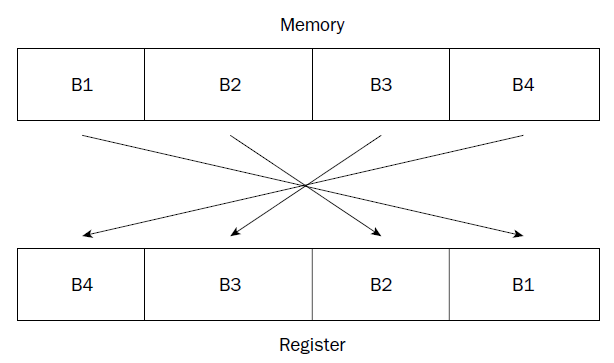(gdb) x/x &data
0x80490bc : 0x00000225
(gdb) x/4b &data
0x80490bc : 0x25 0x02 0x00 0x00
(gdb) print/x $eax # reminding that ' print ' with no /x shows decimal value$1 = 0x225
(gdb)
The decimal value 549 is stored in memory location data, and moved to the EAX register. The first gdb command uses the x command to display the value in memory located at the data label in hexadecimal format. The hexadecimal display shows what we would expect for the hex version of 549. The next command displays the 4 bytes that make up the integer value. Notice that the binary format version shows the 0x25 and 0x02 hex values reversed, which is what we would expect for little-endian format. The last command uses the print command to display the same value after it is loaded into the EAX register, again in hexadecimal format.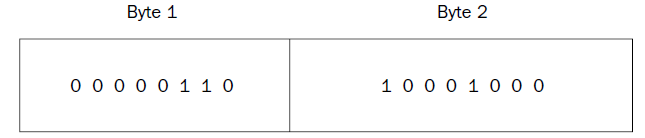= 0x688 = 6*256+8*16+8*1 = 1672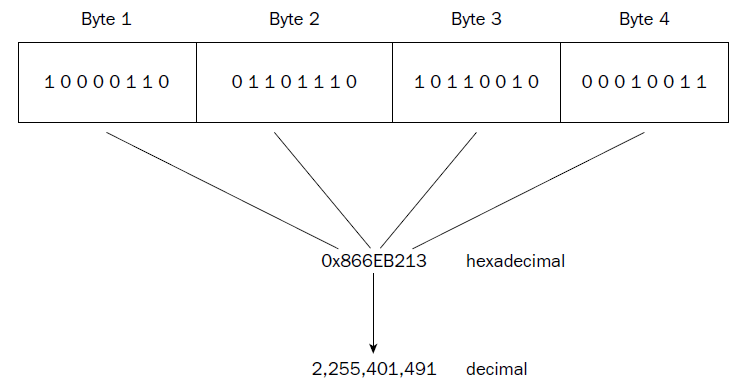each byte is represented by a hexadecimal pair (4 bits per hexadecimal value), which are combined to form the eight-character hexadecimal value. Again, this example uses big-endian format, as seen in the register.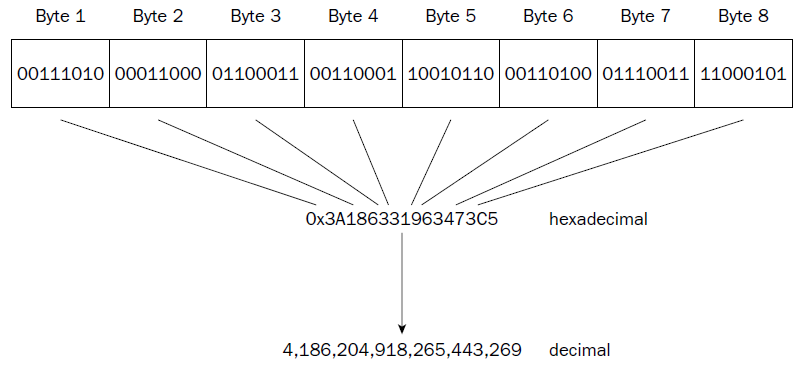❑ Unsigned integers
❑ Signed integers
❑ Binary-coded decimal
❑ Packed binary-coded decimal
❑ Single-precision floating-point
❑ Double-precision floating-point
❑ Double-extended floating-point

the SIMD extensions on Pentium processors add other advanced numeric data types:
❑ 64-bit packed integers
❑ 128-bit packed integers
❑ 128-bit packed single-precision floating-point
❑ 128-bit packed double-precision floating-point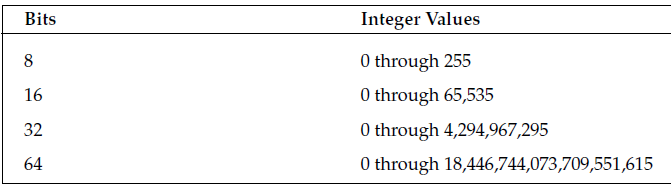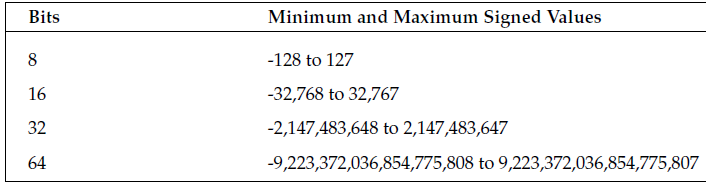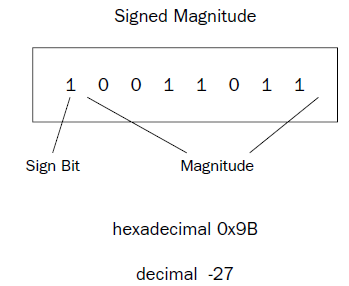One problem with the signed magnitude method is that there are two different ways to express a zero value: 00000000 (decimal +0) and 10000000 (decimal -0). This can complicate some mathematical processes. Also, arithmetic using signed magnitude numbers is complicated, as adding and subtracting simple signed integers cannot be done in the same way as unsigned numbers. For example, doing a simple binary addition of the values 00000001 (decimal 1) and 10000001 (decimal –1) produces 10000010 (decimal –2), which is not the correct answer. Different arithmetic instructions for signed integers and unsigned numbers would be required on the processor.

ONE’s complement
The one’s complement method takes the inverse of the unsigned integer value to produce the similar negative value. The inverse changes any zero bits to ones, and any ones bits to zeroes. Thus, the one’s complement of 00000001 would be 11111110. Again, as with signed magnitude numbers, one’s complement numbers have some problems when performing mathematical operations. There are two ways of representing a zero value (00000000 and 11111111), and arithmetic with one’s complement numbers is also complicated (it does not allow you to do standard binary math).

TWO’s complement

The two’s complement method solves the arithmetic problem of the signed magnitude and one’s complement methods using a simple mathematical trick. For negative integer values, a one is added to the one’s complement of the value.
For example, to find the two’s complement value for decimal -1 you would do the following: 1. Take the one’s complement of 00000001, which is 11111110.
2. Add one to the one’s complement, which is 11111111.
Doing the same for the value -2 you would get 11111110, and for -3 it would be 11111101. You may notice a trend here.
The two’s complement value counts down from 11111111 (decimal –1) until it gets to 10000000, which represents -128. Of course, for multibyte integer sizes the same principle applies across the bytes.
While this seems like an odd thing to do, it solves all of the problems in adding and subtracting signed integers. For example, adding the values 00000001 (+1) and 11111111 (-1) produces the value 00000000, along with a carry value.
The carry value is ignored in signed integer arithmetic, so the final value is indeed 0.
The same hardware can be used to add and subtract both unsigned and signed values.

0...........................127.........................255

0+1.......+63.......+127-128........-64.......-1

When converting unsigned integer values to a larger bit size (such as converting a word to a doubleword), you must ensure that all of the leading bits are set to zero. You should not simply copy one value to the other, as shown here:

movw %ax, %bx

There is no guarantee that the upper part of the EBX register contains zeroes. To accomplish this, you must use two instructions:

movl $0, %ebx movw %ax, %ebx The MOVL instruction is used to load a zero value in the EBX register. This guarantees that the EBX register is completely zero. Then you can safely move the unsigned integer value in the AX register to the EBX register. To help you in these situations, Intel provides the MOVZX instruction. This instruction moves a smallersized unsigned integer value (in either a register or a memory location) to a larger-sized unsigned integer value (only in a register). The format of the MOVZX instruction is movzx source, destination where source can be an 8-bit or 16-bit register or memory location, and destination can be a 16-bit or 32-bit register. The movzxtest.s program demonstrates this instruction: # movzxtest.s .section .text .globl _start _start: nop movl$279, %ecx
movzx %cl, %ebx ########## ecl , ech = WRONG

// CX = 100010111 = 279
// CH = 00000001 = 1
// CL = 00010111 = 23

movl $1, %eax int$0x80

The movzxtest.s program simply puts a large value in the ECX register, and then uses the MOVZX instruction to copy the lower 8 bits to the EBX register. Because the value placed in the ECX register used a word unsigned integer to represent it (it is larger than 255), the value in CL represents only part of the complete value. You can watch the program in the debugger and see what is happening to the registers:

$gdb -q movzxtest (gdb) break *_start+1 Breakpoint 1 at 0x8048075: file movzxtest.s, line 5. (gdb) run Starting program: /home/rich/palp/chap07/movzxtest Breakpoint 1, _start () at movzxtest.s:5 5 movl$279, %ecx
Current language: auto; currently asm
(gdb) s
6 movzx %cl, %ebx
(gdb) s
7 movl $1, %eax (gdb) print$ecx
$1 = 279 (gdb) print$ebx
$2 = 23 (gdb) print/x$ecx
$3 = 0x117 (gdb) print/x$ebx
$4 = 0x17 (gdb) By printing out the decimal values of the EBX and ECX registers, you can tell right away that the unsigned integer value was not copied correctly—the original value was 279 but the new value is only 23. By displaying the values in hexadecimal, you can see what happened. The original value in hex is 0x0117, which takes a doubleword to hold. The MOVZX instruction moved just the lower byte of the ECX register, but zeroed out the remaining bytes in the EBX register, producing the 0x17 value in the EBX register. # ch .section .text .globl _start _start: nop movl$279, %ecx

movzx %ch, %ebx ############## 00000001

movl $1, %eax int$0x80

C:\>gdb -q users\rnz\desktop\ch.exe
(gdb) break *_start+1

(gdb) run

(gdb) print $ebx$1 = 1
(gdb) print $ch$2 = 1
(gdb) print $ech$3 = void
(gdb)
(gdb) print $cx$4 = 279
(gdb) print/x $ecx$5 = 0x117
(gdb) print/x $ebx$6 = 0x1
(gdb) print $cl$1 = 23
(gdb) print $ecx$4 = 279
(gdb) print $ecl$5 = void

Extending signed integer values is different than extending unsigned integers. Padding the high bits with zeroes will change the value of the data for negative numbers. For example, the value -1 (11111111) moved to a doubleword would yield the value 0000000011111111, which in signed integer notation would be +127, not -1. For a signed extension to work, the new bits added must be set to a one value. Thus, the new doubleword would yield the value 1111111111111111, which is the signed integer notation for the value -1, which is what it should be.
Intel has provided the MOVSX instruction to allow extending signed integers and preserving the sign. It is similar to the MOVSZ instruction, but it assumes that the bytes to be moved are in signed integer format and attempts to preserve the signed integer value for the move.

# _movsx
.section .text
.globl _start
_start:
nop
movw $-79, %cx movl$0, %ebx
movw %cx, %bx
movsx %cx, %eax
movl $1, %eax movl$0, %ebx
int $0x80 # _movsx in WINDOWS using MINGW-W64 .section .text //.globl _start /*ENTRY POINT */ //_start: /*ENTRY POINT*/ nop movw$-79, %cx
movl $0, %ebx movw %cx, %bx movsx %cx, %eax movl$1, %eax
movl $0, %ebx int$0x21

//gdb break 1
//*_start+1 not necessary

5 nop
(gdb) s
6 movw $-79, %cx (gdb) s 7 movl$0, %ebx
(gdb) s
8 movw %cx, %bx
(gdb) s
9 movsx %cx, %eax
(gdb) info reg
eax 0x60ffcc 6356940
ecx 0x40ffb1 4259761
edx 0x401000 4198400
ebx 0xffb1 65457
esp 0x60ff74 0x60ff74
ebp 0x60ff80 0x60ff80
esi 0x401000 4198400
edi 0x401000 4198400
eip 0x40100d 0x40100d
eflags 0x246 [ PF ZF IF ]
cs 0x23 35
ss 0x2b 43
ds 0x2b 43
es 0x2b 43
fs 0x53 83
gs 0x2b 43
(gdb) print $bx$1 = -79
(gdb) print $cx$2 = -79
(gdb)

# mmovsx
.section .text
.globl _start
_start:
nop
movw $79, %cx xor %ebx, %ebx ############# set to zero in every case movw %cx, %bx movsx %cx, %eax movl$1, %eax
movl $0, %ebx int$0x80

.section .data
data1:
.int 1, -1, 463345, -333252322, 0 # every element of array has 4 bytes
data2:
.quad 1, -1, 463345, -333252322, 0 # every element has 8 bytes
.section .text
.globl _start
_start:
nop
movl $1, %eax movl$0, %ebx
int $0x21 gdb -q users\rnz\desktop\qquad.exe (gdb) break 1 (gdb) run Starting program: C:\users\rnz\desktop\qquad.exe [New Thread 440.0x260] Breakpoint 1, start () at users\rnz\desktop\qquad.s:10 10 nop (gdb) x/20b &data1 0x402000 : 0x01 0x00 0x00 0x00 0xff 0xff 0xff 0xff 0x402008 : 0xf1 0x11 0x07 0x00 0x1e 0xf9 0x22 0xec 0x402010 : 0x00 0x00 0x00 0x00 //32 byte each element (gdb) (gdb) x/40b &data2 0x402014 : 0x01 0x00 0x00 0x00 0x00 0x00 0x00 0x00 0x40201c : 0xff 0xff 0xff 0xff 0xff 0xff 0xff 0xff 0x402024 : 0xf1 0x11 0x07 0x00 0x00 0x00 0x00 0x00 0x40202c : 0x1e 0xf9 0x22 0xec 0xff 0xff 0xff 0xff 0x402034 : 0x00 0x00 0x00 0x00 0x00 0x00 0x00 0x00 //64 bit each element (gdb) (gdb) x/5d &data1 0x402000 : 1 -1 463345 -333252322 0x402010 : 0 (gdb) 0x402014 : 1 0 -1 -1 0x402024 : 463345 //WRONG , x/d NOT SUFFICIENT. the debugger didn’t know how to handle displaying the values using just the x/d command. If you want to display quadword signed integer values in the debugger, you must use the gd option (gdb) (gdb) x/5gd &data2 0x402014 : 1 -1 0x402024 : 463345 -333252322 0x402034 : 0 (gdb) SIMD Integers The Intel Single Instruction Multiple Data (SIMD) technology provides additional ways to define integers These new integer types enable the processor to perform arithmetic operations on a group of multiple integers simultaneously. The SIMD architecture uses the packed integer data type. A packed integer is a series of bytes that can represent more than one integer value. Mathematical operations can be performed on the series of bytes as a whole, working on the individual integer values within the series in parallel. MMX integers The Multimedia Extension (MMX) technology introduced in the Pentium MMX and Pentium II processors provided three new integer types: ❑ 64-bit packed byte integers ❑ 64-bit packed word integers ❑ 64-bit packed doubleword integers Each of these data types provides for multiple integer data elements to be contained (or packed) in a single 64-bit MMX register.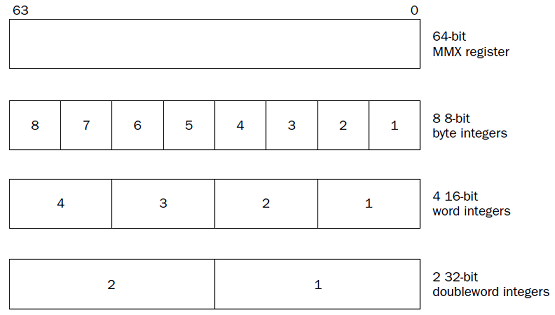the MMX registers are mapped to the FPU registers. we need to save any data stored in the FPU registers in memory before using any MMX register instructions. we can use the MOVQ instruction to move data into an MMX register, but we must decide which of the three packed integer formats . The format of the MOVQ instruction is movq source, destination where source and destination can be an MMX register, an SSE register, or a 64-bit memory location (although you cannot move MMX integers between memory locations). # mmxt .section .data values1: .int 1, -1 # int = 32 bit = double word values2: .byte 0x10, 0x05, 0xff, 0x32, 0x47, 0xe4, 0x00, 0x01 #HEX .section .text .globl _start _start: nop movq values1, %mm0 movq values2, %mm1 movl$1, %eax
movl $0, %ebx //int$0x80 in Linux
int $0x21 (gdb) print$mm0
$1 = {uint64 = -4294967295, v2_int32 = {1, -1}, v4_int16 = {1, 0, -1, -1}, v8_int8 = {1, 0, 0, 0, -1, -1, -1, -1}} (gdb) print/t$mm0
$3 = {uint64 = 1111111111111111111111111111111100000000000000000000000000000001, v2_int32 = {1, 11111111111111111111111111111111}, v4_int16 = {1, 0, 1111111111111111, 1111111111111111}, v8_int8 = {1, 0, 0, 0, 11111111, 11111111, 11111111, 11111111}} (gdb) (gdb) print/x$mm0
$2 = {uint64 = 0xffffffff00000001, v2_int32 = {0x1, 0xffffffff}, v4_int16 = {0x1, 0x0, 0xffff, 0xffff}, v8_int8 = {0x1, 0x0, 0x0, 0x0, 0xff, 0xff, 0xff, 0xff}} (gdb) print/x$mm1
$3 = {uint64 = 0x100e44732ff0510, v2_int32 = {0x32ff0510, 0x100e447}, v4_int16 = {0x510, 0x32ff, 0xe447, 0x100}, v8_int8 = {0x10, 0x5, 0xff, 0x32, 0x47, 0xe4, 0x0, 0x1}} # mmxtd .section .data values1: .int 1, -1 # int = 32 bit = double word values2: .byte 16, 5, 255, 50, 71, 228, 0, 1 #DEC .section .text .globl _start _start: nop movq values1, %mm0 movq values2, %mm1 movl$1, %eax
movl $0, %ebx //int$0x80 in Linux
int $0x21 C:\>as -gstabs -o users\rnz\desktop\xmtd.o users\rnz\desktop\xmtd.s Assembler messages: Error: can't open users\rnz\desktop\xmtd.s for reading: No such file or directory C:\>as -gstabs -o users\rnz\desktop\mmxtd.o users\rnz\desktop\mmxtd.s C:\>ld -o users\rnz\desktop\mmxtd.exe users\rnz\desktop\mmxtd.o C:\>gdb -q users\rnz\desktop\mmxtd.exe Reading symbols from users\rnz\desktop\mmxtd.exe...done. (gdb) break 1 # in linux break *_start+1 Breakpoint 1 at 0x401000: file users\rnz\desktop\mmxtd.s, line 1. (gdb) run Starting program: C:\users\rnz\desktop\mmxtd.exe [New Thread 7976.0x36d4] Breakpoint 1, start () at users\rnz\desktop\mmxtd.s:10 10 nop (gdb) s 11 movq values1, %mm0 (gdb) s 12 movq values2, %mm1 (gdb) s 13 movl$1, %eax
(gdb) s
14 movl $0, %ebx (gdb) s 16 int$0x21
(gdb) print $mm0$1 = {uint64 = -4294967295, v2_int32 = {1, -1}, v4_int16 = {1, 0, -1, -1}, v8_int8 = {1, 0, 0, 0, -1, -1, -1, -1}}
(gdb) print/x $mm0$5 = {uint64 = 0xffffffff00000001, v2_int32 = {0x1, 0xffffffff}, v4_int16 = {0x1, 0x0, 0xffff, 0xffff}, v8_int8 = {0x1, 0x0, 0x0, 0x0, 0xff, 0xff, 0xff, 0xff}}
(gdb) print $mm1$3 = {uint64 = 72308588487312656, v2_int32 = {855573776, 16835655}, v4_int16 = {1296, 13055, -7097, 256}, v8_int8 = {16, 5, -1, 50, 71, -28, 0, 1}}
(gdb) print/x $mm1$4 = {uint64 = 0x100e44732ff0510, v2_int32 = {0x32ff0510, 0x100e447}, v4_int16 = {0x510, 0x32ff, 0xe447, 0x100}, v8_int8 = {0x10, 0x5, 0xff, 0x32, 0x47, 0xe4, 0x0, 0x1}}

(gdb) print/f $mm1$21 = {uint64 = 7.697470564745318e-304, v2_int32 = {2.96882092e-08, 2.3673668e-38}, v4_int16 = {1296, 13055, -7097, 256}, v8_int8 = {16, 5, -1, 50, 71, -28, 0, 1}}
(gdb) print/f $mm0$22 = {uint64 = -nan(0xfffff00000001), v2_int32 = {1.40129846e-45, -nan(0x7fffff)}, v4_int16 = {1, 0, -1, -1}, v8_int8 = {1, 0, 0, 0, -1, -1, -1, -1}}
(gdb)

// STXR are the FPU Registers counterparts of MMX
// mm0 register is mapped to the first FPU register, st0r
// the same for the other 7 registers

SSE integers

The Streaming SIMD Extensions (SSE) technology (also described in Chapter 2) provides eight 128-bit
XMM registers (named XMM0 through XMM7) for handling packed data. The SSE2 technology (introduced in the Pentium 4 processor) provides four additional packed signed integer data types:

❑ 128-bit packed byte integers
❑ 128-bit packed word integers
❑ 128-bit packed doubleword integers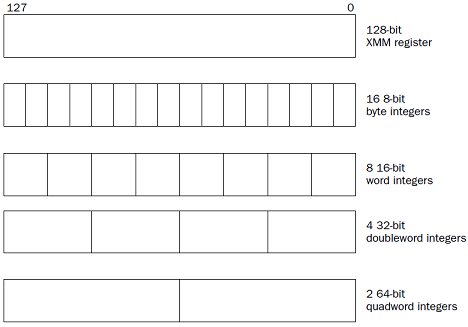# xmm01
.section .data
valu0:
.int 1, -1, 0, 135246 # 128 bit
valu1:
.quad 1, -1 # 128 bit
.section .text
//.globl _start
//_start:
nop
movdqu valu0, %xmm0
movdqu valu1, %xmm1
movl $1, %eax movl$0, %ebx
//int $0x80 int$0x21

C:\>as -gstabs -o users\rnz\desktop\xmm01.o users\rnz\desktop\xmm01.s
C:\>ld -o users\rnz\desktop\xmm01.exe users\rnz\desktop\xmm01.o
C:\>gdb -q users\rnz\desktop\xmm01.exe
(gdb) break 1
Breakpoint 1 at 0x401000: file users\rnz\desktop\xmm01.s, line 1.
(gdb) run
Starting program: C:\users\rnz\desktop\xmm01.exe
Breakpoint 1, ?? () at users\rnz\desktop\xmm01.s:10
10 nop
(gdb) s
11 movdqu valu0, %xmm0
(gdb) s
12 movdqu valu1, %xmm1
(gdb) s
13 movl $1, %eax (gdb) s 14 movl$0, %ebx
(gdb) s
16 int $0x21 (gdb) print$xmm0
$1 = {v4_float = {1.40129846e-45, -nan(0x7fffff), 0, 1.89520012e-40}, v2_double = {-nan(0xfffff00000001), 2.8699144274488922e-309}, v16_int8 = {1, 0, 0, 0, -1, -1, -1, -1, 0, 0, 0, 0, 78, 16, 2, 0}, v8_int16 = {1, 0, -1, -1, 0, 0, 4174, 2}, v4_int32 = {1, -1, 0, 135246}, v2_int64 = {-4294967295, 580877146914816}, uint128 = 10715292067404213048920514521726977} (gdb) print/x$xmm0
$2 = {v4_float = {0x0, 0x0, 0x0, 0x0}, v2_double = {0x8000000000000000, 0x0}, v16_int8 = {0x1, 0x0, 0x0, 0x0, 0xff, 0xff, 0xff, 0xff, 0x0, 0x0, 0x0, 0x0, 0x4e, 0x10, 0x2, 0x0}, v8_int16 = {0x1, 0x0, 0xffff, 0xffff, 0x0, 0x0, 0x104e, 0x2}, v4_int32 = {0x1, 0xffffffff, 0x0, 0x2104e}, v2_int64 = {0xffffffff00000001, 0x2104e00000000}, uint128 = 0x2104e00000000ffffffff00000001} (gdb) print/t$xmm0
$3 = {v4_float = {0, 0, 0, 0}, v2_double = {1000000000000000000000000000000000000000000000000000000000000000, 0}, v16_int8 = {1, 0, 0, 0, 11111111, 11111111, 11111111, 11111111, 0, 0, 0, 0, 1001110, 10000, 10, 0}, v8_int16 = {1, 0, 1111111111111111, 1111111111111111, 0, 0, 1000001001110, 10}, v4_int32 = {1, 11111111111111111111111111111111, 0, 100001000001001110}, v2_int64 = {1111111111111111111111111111111100000000000000000000000000000001, 10000100000100111000000000000000000000000000000000}, uint128 = 100001000001001110000000000000000000000000000000001111111111111111111111111111111100000000000000000000000000000001} (gdb) print/f$xmm0
$4 = {v4_float = {1.40129846e-45, -nan(0x7fffff), 0, 1.89520012e-40}, v2_double = {-nan(0xfffff00000001), 2.8699144274488922e-309}, v16_int8 = {1, 0, 0, 0, -1, -1, -1, -1, 0, 0, 0, 0, 78, 16, 2, 0}, v8_int16 = {1, 0, -1, -1, 0, 0, 4174, 2}, v4_int32 = {1.40129846e-45, -nan(0x7fffff), 0, 1.89520012e-40}, v2_int64 = { -nan(0xfffff00000001), 2.8699144274488922e-309}, uint128 = 10715292067404213048920514521726977} (gdb) print$xmm1
$5 = {v4_float = {1.40129846e-45, 0, -nan(0x7fffff), -nan(0x7fffff)}, v2_double = {4.9406564584124654e-324, -nan(0xfffffffffffff)}, v16_int8 = {1, 0, 0, 0, 0, 0, 0, 0, -1, -1, -1, -1, -1, -1, -1, -1}, v8_int16 = {1, 0, 0, 0, -1, -1, -1, -1}, v4_int32 = {1, 0, -1, -1}, v2_int64 = {1, -1}, uint128 = 340282366920938463444927863358058659841} (gdb) print/x$xmm1
$6 = {v4_float = {0x0, 0x0, 0x0, 0x0}, v2_double = {0x0, 0x8000000000000000}, v16_int8 = {0x1, 0x0, 0x0, 0x0, 0x0, 0x0, 0x0, 0x0, 0xff, 0xff, 0xff, 0xff, 0xff, 0xff, 0xff, 0xff}, v8_int16 = {0x1, 0x0, 0x0, 0x0, 0xffff, 0xffff, 0xffff, 0xffff}, v4_int32 = {0x1, 0x0, 0xffffffff, 0xffffffff}, v2_int64 = {0x1, 0xffffffffffffffff}, uint128 = 0xffffffffffffffff0000000000000001} (gdb) print/t$xmm1
$7 = {v4_float = {0, 0, 0, 0}, v2_double = {0, 1000000000000000000000000000000000000000000000000000000000000000}, v16_int8 = {1, 0, 0, 0, 0, 0, 0, 0, 11111111, 11111111, 11111111, 11111111, 11111111, 11111111, 11111111, 11111111}, v8_int16 = {1, 0, 0, 0, 1111111111111111, 1111111111111111, 1111111111111111, 1111111111111111}, v4_int32 = {1, 0, 11111111111111111111111111111111, 11111111111111111111111111111111}, v2_int64 = {1, 1111111111111111111111111111111111111111111111111111111111111111}, uint128 = 11111111111111111111111111111111111111111111111111111111111111110000000000000000000000000000000000000000000000000000000000000001} (gdb) print/f$xmm1
$8 = {v4_float = {1.40129846e-45, 0, -nan(0x7fffff), -nan(0x7fffff)}, v2_double = {4.9406564584124654e-324, -nan(0xfffffffffffff)}, v16_int8 = {1, 0, 0, 0, 0, 0, 0, 0, -1, -1, -1, -1, -1, -1, -1, -1}, v8_int16 = {1, 0, 0, 0, -1, -1, -1, -1}, v4_int32 = {1.40129846e-45, 0, -nan(0x7fffff), -nan(0x7fffff)}, v2_int64 = { 4.9406564584124654e-324, -nan(0xfffffffffffff)}, uint128 = 340282366920938463444927863358058659841} (gdb) Each BCD value is an unsigned 8-bit integer, with a value range of 0 to 9. The 8-bit values higher than 9 are considered invalid in BCD. Bytes containing BCD values are combined to represent decimal digits.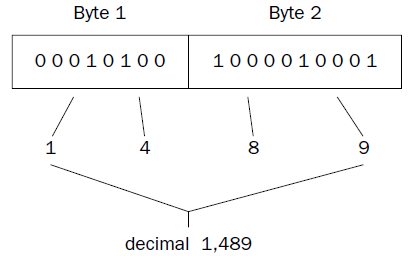FPU BCD values The FPU registers can be used for BCD arithmetic operations within the FPU. The FPU contains eight 80- bit registers (ST0 through ST7), which can also be used to hold 80-bit BCD values. The BCD values are stored using the lower 9 bytes, using packed BCD format, two BCD values per byte (producing 18 BCD digits). The last byte of the FPU register is mostly unused, with the exception of the highest-order bit. This bit is used as a sign indicator ' 1 ' indicates a negative BCD value, and a ' 0 ' indicates a positive value.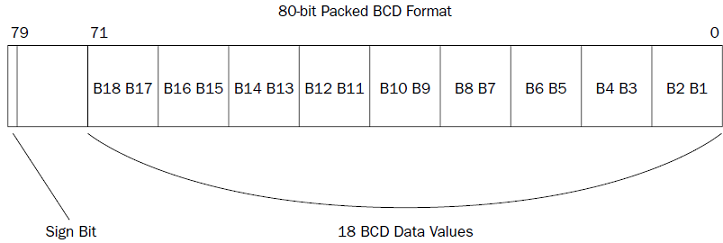fbld source where source is an 80-bit memory location. # bcdtest .section .data data1: .byte 0x34, 0x12, 0x00, 0x00, 0x00, 0x00, 0x00, 0x00, 0x00, 0x00 data2: .int 2 .section .text .globl _start _start: nop fbld data1 fimul data2 fbstp data1 movl$1, %eax
movl $0, %ebx int$0x80

This program creates a simple BCD value representing the decimal value 1,234 at the memory location defined by the label data1 (remember that Intel uses little-endian notation).
The FBLD instruction is used to load the value into the top of the FPU register stack (ST0).
The FIMUL instruction is used to multiply the ST0 register by the integer value at the data2 memory location.
Finally, the FBSTP instruction is used to move the new value on the stack back into the data1 memory location.

(gdb) x/10b &data1
0x8049094 : 0x34 0x12 0x00 0x00 0x00 0x00 0x00 0x00
0x804909c : 0x00 0x00
The BCD value for 1,234 is loaded at the data1 memory location. Next, step through the FBLD instruction, and check the value in the ST0 register using the info all command:
(gdb)
12 fimul data2

(gdb) info all # INFO ALL

st0 1234 (raw 0x40099a40000000000000)

When you find the ST0 register value in the list of registers, it should show that it is loaded with the decimal value 1,234. You may notice, however, that the hexadecimal value of the register is not in 80-bit packed BCD format. Remember that the BCD value is converted to the floating-point representation while in the FPU.
Now step through the next instruction (FIMUL) and view the registers again:
(gdb) s
13 fbstp data1
(gdb) info all # INFO ALL
st0 2468 (raw 0x400a9a40000000000000)
Indeed, the value in the ST0 register was multiplied by 2. The last step should place the value in ST0 back into the data1 memory location. You can check that by displaying the memory location:
(gdb) x/10b &data1
0x8049094 : 0x68 0x24 0x00 0x00 0x00 0x00 0x00 0x00
0x804909c : 0x00 0x00
new value was placed in the data1 memory location, back in BCD format.